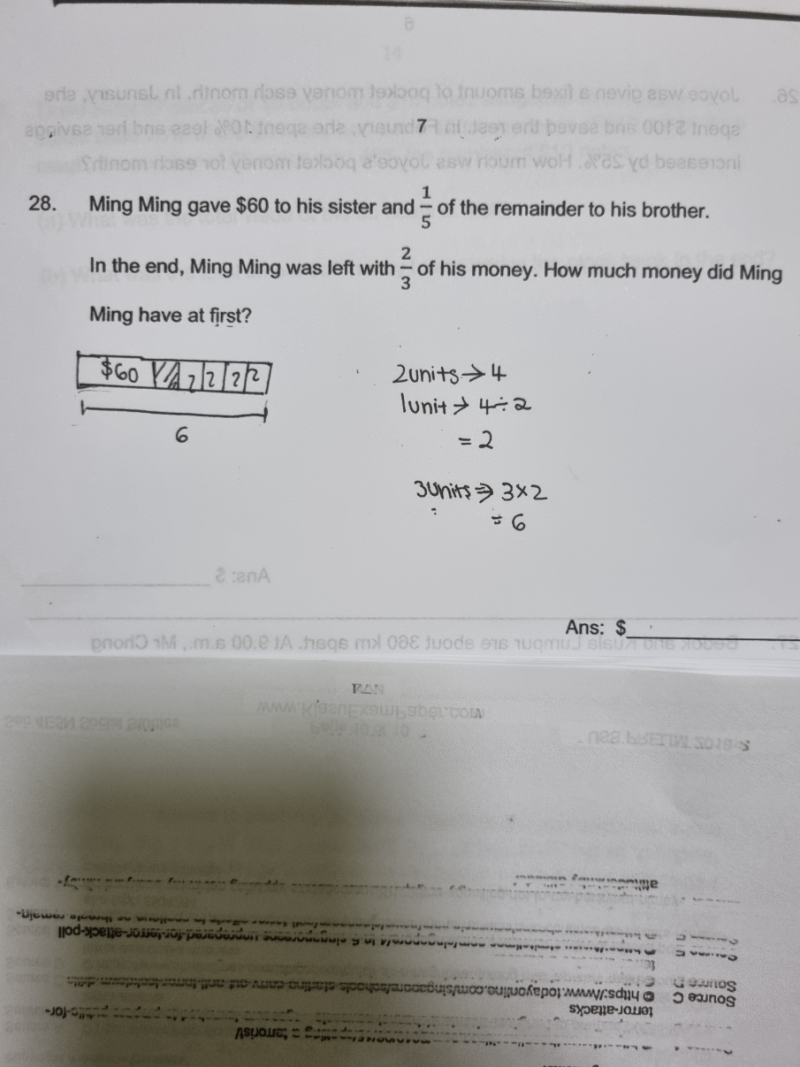# QuestionDo till here .confuse how to continue.thank you.

Solution 1:

Given away      Remainder            Total

[u]                  [u][u][u][u]              60+5u

4u —> (2/3) (60+ 5u)

or

60 + u  =  (1/3)(60+5u)

180 + 3u = 60 + 5u

2u = 120

u =60

60 + 5u = 60 + 300 = 360

Hence Ming Ming has \$360 at first.

Solution 2:

Alternatively,  continuing from your working….

2 part of money —> 4u

1 part of money —> 4u/2 = 2u    =>  Total = 6u

So if   60 + u = 2u  => u = 60

60 x 6 = 360

Hence Ming Ming has \$360 at first.

PS:  If you find Solution 2 confusing, maybe Solution 1 is easier to understand?